# Shape

This class allows creating interconnected graphical elements on a PDF page. Its methods have the same meaning and name as the corresponding Page methods. Their Common Parameters are however exported to a separate method, `finish()`. In addition, all draw methods return a Point object to support connected drawing paths. This point always equals the “current point”, that PDF maintains during path construction.

The class now also supports the text insertion methods `insertText()` and `insertTextbox()`. They need a slightly different handling compared to the draw methods (see below for details):

1. They do not use `Shape.contents`. Instead they directly modify `Shape.totalcont`.
2. They do not use nor need `Shape.finish()`.
3. They provide their own `color` and `morph` arguments.
4. They do not use nor change `Shape.lastPoint`.

As with the draw methods, text insertion requires using `Shape.commit()` to update the page.

Method / Attribute Description
`Shape.commit()` update the page’s `/Contents` object
`Shape.drawBezier()` draw a cubic Bézier curve
`Shape.drawCircle()` draw a circle around a point
`Shape.drawCurve()` draw a cubic Bézier using one helper point
`Shape.drawLine()` draw a line
`Shape.drawOval()` draw an ellipse
`Shape.drawPolyline()` connect a sequence of points
`Shape.drawRect()` draw a rectangle
`Shape.drawSector()` draw a circular sector or piece of pie
`Shape.drawSquiggle()` draw a squiggly line
`Shape.drawZigzag()` draw a zigzag line
`Shape.finish()` finish a set of draws
`Shape.insertText()` insert text lines
`Shape.insertTextbox()` insert text into a rectangle
`Shape.contents` draw commands since last `finish()`
`Shape.doc` stores the page’s document
`Shape.height` stores the page’s height
`Shape.lastPoint` stores the current point
`Shape.page` stores the owning page
`Shape.width` stores the page’s width
`Shape.totalcont` accumulated string to be stored in `/Contents`

Class API

class `Shape`
`__init__`(self, page)

Create a new drawing. During importing PyMuPDF, the `fitz.Page` object is being given the convenience method `newShape()` to construct a `Shape` object. During instantiation, a check will be made whether we do have a PDF page. An exception is otherwise raised.

Parameters: page (Page) – an existing page of a PDF document.
`drawLine`(p1, p2)

Draw a line from Point objects `p1` to `p2`.

Parameters: p1 (Point) – starting point p2 (Point) – end point Point the end point, `p2`.
`drawSquiggle`(p1, p2, breadth = 2)

Draw a squiggly (wavy, undulated) line from Point objects `p1` to `p2`. An integer number of full wave periods will always be drawn, one period having a length of `4 * breadth`. The breadth parameter will be adjusted as necessary to meet this condition. The drawn line will always turn “left” when leaving `p1` and always join `p2` from the “right”.

Parameters: p1 (Point) – starting point p2 (Point) – end point breadth (float) – the amplitude of each wave. The condition `2 * breadth < abs(p2 - p1)` must be true to fit in at least one wave. See the following picture, which shows two points connected by one full period. Point the end point, `p2`.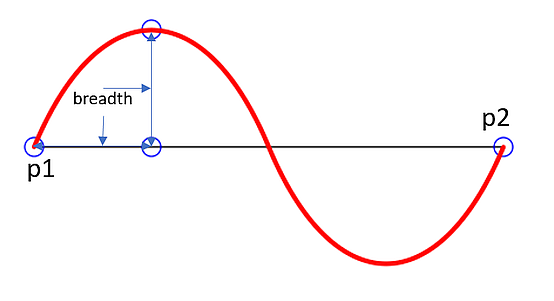Here is an example of three connected lines, forming a closed, filled triangle. Little arrows indicate the stroking direction.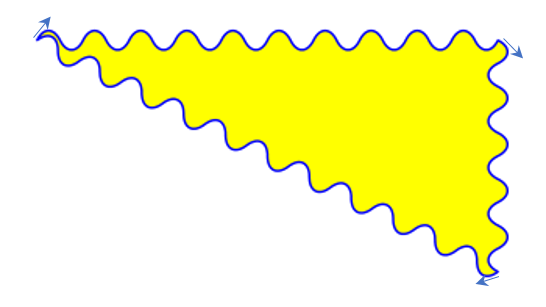Note

Waves drawn are not trigonometric (sine / cosine). If you need that, have a look at draw-sines.py.

`drawZigzag`(p1, p2, breadth = 2)

Draw a zigzag line from Point objects `p1` to `p2`. An integer number of full zigzag periods will always be drawn, one period having a length of `4 * breadth`. The breadth parameter will be adjusted to meet this condition. The drawn line will always turn “left” when leaving `p1` and always join `p2` from the “right”.

Parameters: p1 (Point) – starting point p2 (Point) – end point breadth (float) – the amplitude of the movement. The condition `2 * breadth < abs(p2 - p1)` must be true to fit in at least one period. Point the end point, `p2`.
`drawPolyline`(points)

Draw several connected lines between points contained in the sequence `points`. This can be used for creating arbitrary polygons by setting the last item equal to the first one.

Parameters: points (sequence) – a sequence of Point objects. Its length must at least be 2 (in which case it is equivalent to `drawLine()`). Point `points[-1]` - the last point in the argument sequence.
`drawBezier`(p1, p2, p3, p4)

Draw a standard cubic Bézier curve from `p1` to `p4`, using `p2` and `p3` as control points.

Parameters: p1 (Point) – starting point p2 (Point) – control point 1 p3 (Point) – control point 2 p4 (Point) – end point Point the end point, `p4`.

Example: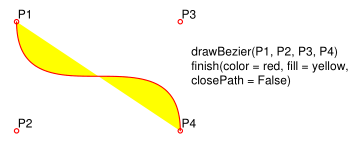`drawOval`(rect)

Draw an ellipse inside the given rectangle. If `rect` is a square, a standard circle is drawn. The drawing starts and ends at the middle point of the left rectangle side in a counter-clockwise movement.

Parameters: rect (Rect) – rectangle, must be finite and not empty. Point the middle point of the left rectangle side.
`drawCircle`(center, radius)

Draw a circle given its center and radius. The drawing starts and ends at point `start = center - (radius, 0)` in a counter-clockwise movement. `start` corresponds to the middle point of the enclosing square’s left border.

The method is a shortcut for `drawSector(center, start, 360, fullSector = False)`. To draw a circle in a clockwise movement, change the sign of the degree.

Parameters: center (Point) – the center of the circle. radius (float) – the radius of the circle. Must be positive. Point `center - (radius, 0)`.
`drawCurve`(p1, p2, p3)

A special case of `drawBezier()`: Draw a cubic Bézier curve from `p1` to `p3`. On each of the two lines from `p1` to `p2` and from `p2` to `p3` one control point is generated. This guaranties that the curve’s curvature does not change its sign. If these two connecting lines intersect with an angle of 90 degress, then the resulting curve is a quarter ellipse (or quarter circle, if of same length) circumference.

Parameters: p1 (Point) – starting point. p2 (Point) – helper point. p3 (Point) – end point. Point the end point, `p3`.

Example: a filled quarter ellipse segment.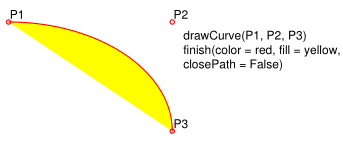`drawSector`(center, point, angle, fullSector = True)

Draw a circular sector, optionally connecting the arc to the circle’s center (like a piece of pie).

Parameters: center (Point) – the center of the circle. point (Point) – one of the two end points of the pie’s arc segment. The other one is calculated from the `angle`. angle (float) – the angle of the sector in degrees. Used to calculate the other end point of the arc. Depending on its sign, the arc is drawn counter-clockwise (postive) or clockwise. fullSector (bool) – whether to draw connecting lines from the ends of the arc to the circle center. If a fill color is specified, the full “pie” is colored, otherwise just the sector. the other end point of the arc. Can be used as starting point for a following invocation to create logically connected pies charts. Point

Examples: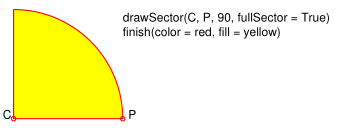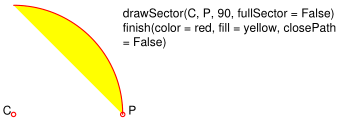`drawRect`(rect)

Draw a rectangle. The drawing starts and ends at the top-left corner in a counter-clockwise movement.

Parameters: rect (Rect) – where to put the rectangle on the page. Point `rect.top_left` (top-left corner of the rectangle).
`insertText`(point, text, fontsize = 11, fontname = "Helvetica", fontfile = None, idx = 0, set_simple = False, color = (0, 0, 0), rotate = 0, morph = None)

Insert text lines beginning at a Point `point`.

Parameters: point (Point) – the bottom-left position of the first `text` character in pixels. `point.x` specifies the distance from left border, `point.y` the distance from top of page. This is independent from text orientation as requested by `rotate`. However, there must always be sufficient room “above”, which can mean the distance from any of the four page borders. text (str or sequence) – the text to be inserted. May be specified as either a string type or as a sequence type. For sequences, or strings containing line breaks `\n`, several lines will be inserted. No care will be taken if lines are too wide, but the number of inserted lines will be limited by “vertical” space on the page (in the sense of reading direction as established by the `rotate` parameter). Any rest of `text` is discarded - the return code however contains the number of inserted lines. Only single byte character codes are currently supported. rotate (int) – determines whether to rotate the text. Acceptable values are multiples of 90 degrees. Default is 0 (no rotation), meaning horizontal text lines oriented from left to right. 180 means text is shown upside down from right to left. 90 means counter-clockwise rotation, text running upwards. 270 (or -90) means clockwise rotation, text running downwards. In any case, `point` specifies the bottom-left coordinates of the first character’s rectangle. Multiple lines, if present, always follow the reading direction established by this parameter. So line 2 is located above line 1 in case of `rotate = 180`, etc. int number of lines inserted.

For a description of the other parameters see Common Parameters.

`insertTextbox`(rect, buffer, fontsize = 11, fontname = "Helvetica", fontfile = None, idx = 0, set_simple = False, color = (0, 0, 0), expandtabs = 8, align = TEXT_ALIGN_LEFT, rotate = 0, morph = None)

PDF only: Insert text into the specified rectangle. The text will be split into lines and words and then filled into the available space, starting from one of the four rectangle corners, depending on `rotate`. Line feeds will be respected as well as multiple spaces will be.

Parameters: rect (Rect) – the area to use. It must be finite and not empty. buffer – the text to be inserted. Must be specified as a string or a sequence of strings. Line breaks are respected also when occurring in a sequence entry. align (int) – align each text line. Default is 0 (left). Centered, right and justified are the other supported options, see Text Alignment. Please note that the effect of parameter value `TEXT_ALIGN_JUSTIFY` is only achievable with “simple” (single-byte) fonts (including the PDF Base 14 Fonts). Refer to Adobe PDF Reference 1.7, section 5.2.2, page 399. expandtabs (int) – controls handling of tab characters `\t` using the `string.expandtabs()` method per each line. rotate (int) – requests text to be rotated in the rectangle. This value must be a multiple of 90 degrees. Default is 0 (no rotation). Effectively, four different values are processed: 0, 90, 180 and 270 (= -90), each causing the text to start in a different rectangle corner. Bottom-left is 90, bottom-right is 180, and -90 / 270 is top-right. See the example how text is filled in a rectangle. This argument takes precedence over morphing. See the second example, which shows text first rotated left by 90 degrees and then the whole rectangle rotated clockwise around is lower left corner. float If positive or zero: successful execution. The value returned is the unused rectangle line space in pixels. This may safely be ignored - or be used to optimize the rectangle, position subsequent items, etc. If negative: no execution. The value returned is the space deficit to store text lines. Enlarge rectangle, decrease `fontsize`, decrease text amount, etc.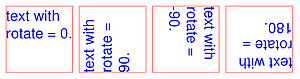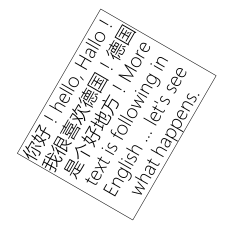For a description of the other parameters see Common Parameters.

`finish`(width = 1, color = (0, 0, 0), fill = None, roundCap = True, dashes = None, closePath = True, even_odd = False, morph = (pivot, matrix))

Finish a set of `draw*()` methods by applying Common Parameters to all of them. This method also supports morphing the resulting compound drawing using a pivotal Point.

Parameters: morph (sequence) – morph the compound drawing around some arbitrary pivotal Point `pivot` by applying Matrix `matrix` to it. Default is no morphing (`None`). The matrix can contain any values in its first 4 components, `matrix.e == matrix.f == 0` must be true, however. This means that any combination of scaling, shearing, rotating, flipping, etc. is possible, but translations are not. even_odd (bool) – request the “even-odd rule” for filling operations. Default is `False`, so that the “nonzero winding number rule” is used. These rules are alternative methods to apply the fill color where areas overlap. Only with fairly complex shapes a different behavior is to be expected with these rules. For an in-depth explanation, see Adobe PDF Reference 1.7, pp. 232 ff. Here is an example to demonstrate the difference.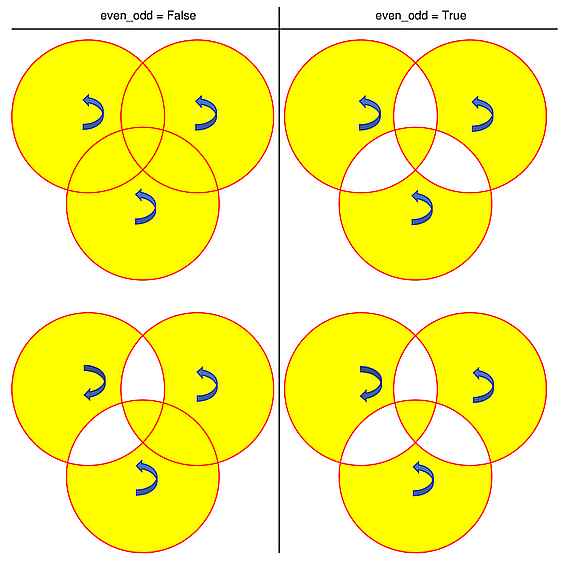Note

Method “even-odd” counts the number of overlaps of areas. Pixels in areas overlapping an odd number of times are regarded inside, otherwise outside. In contrast, the default method “nonzero winding” also looks at the area orientation: it counts `+1` if an area is drawn counter-clockwise and `-1` else. If the result is zero,the pixel is regarded outside, otherwise inside. In the top two shapes, three circles are drawn in standard manner (anti-clockwise, look at the arrows). The lower two shapes contain one (top-left) circle drawn clockwise. As can be seen, area orientation is irrelevant for the even-odd rule.

`commit`(overlay = True)

Update the page’s `/Contents` with the accumulated drawing commands. If a `Shape` is not committed, the page will not be changed. The method must be preceeded with at least one `finish()` or text insertion method.

Parameters: overlay (bool) – determine whether to put the drawing in foreground (default) or background. Relevant only, if the page has a non-empty `/Contents` object.
`doc`

For reference only: the page’s document.

Type: Document
`page`

For reference only: the owning page.

Type: Page
`height`

Copy of the page’s height

Type: float
`width`

Copy of the page’s width.

Type: float
`contents`

Accumulated command buffer for draw methods since last finish.

Type: str
`totalcont`

Total accumulated command buffer for draws and text insertions. This will be used by `Shape.commit()`.

Type: str
`lastPoint`

For reference only: the current point of the drawing path. It is `None` at `Shape` creation and after each `finish()` and `commit()`.

Type: Point

## Usage

A drawing object is constructed by `img = page.newShape()`. After this, as many draw, finish and text insertions methods as required may follow. Each sequence of draws must be finished before the drawing is committed. The overall coding pattern looks like this:

```>>> img = page.newShape()
>>> img.draw1(...)
>>> img.draw2(...)
>>> ...
>>> img.finish(width=..., color = ..., fill = ..., morph = ...)
>>> img.draw3(...)
>>> img.draw4(...)
>>> ...
>>> img.finish(width=..., color = ..., fill = ..., morph = ...)
>>> ...
>>> img.insertText*
>>> ...
>>> img.commit()
>>> ....
```